Important Questions: Exponents and Powers

# Important Questions: Exponents and Powers Notes | Study Mathematics (Maths) Class 8 - Class 8

## Document Description: Important Questions: Exponents and Powers for Class 8 2022 is part of Mathematics (Maths) Class 8 preparation. The notes and questions for Important Questions: Exponents and Powers have been prepared according to the Class 8 exam syllabus. Information about Important Questions: Exponents and Powers covers topics like and Important Questions: Exponents and Powers Example, for Class 8 2022 Exam. Find important definitions, questions, notes, meanings, examples, exercises and tests below for Important Questions: Exponents and Powers.

Introduction of Important Questions: Exponents and Powers in English is available as part of our Mathematics (Maths) Class 8 for Class 8 & Important Questions: Exponents and Powers in Hindi for Mathematics (Maths) Class 8 course. Download more important topics related with notes, lectures and mock test series for Class 8 Exam by signing up for free. Class 8: Important Questions: Exponents and Powers Notes | Study Mathematics (Maths) Class 8 - Class 8
 1 Crore+ students have signed up on EduRev. Have you?

Question 1: Find the value of: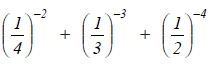Solution: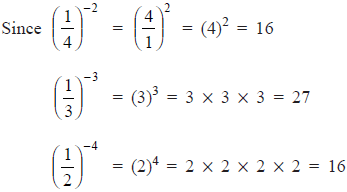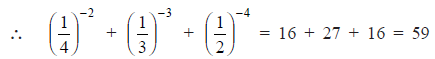Question 2:  Simplify: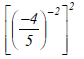Solution: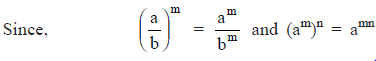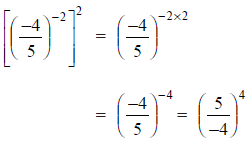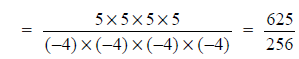Thuss,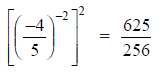Question 3: Find the value of m when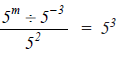Solution: We have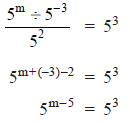Since base is same on both sides, then their exponents are same.
∴ m – 5 = 3
⇒ m = 3 + 5 = 8
Thus, the required value of m is 8.

Question 4: Simplify: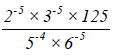Solution: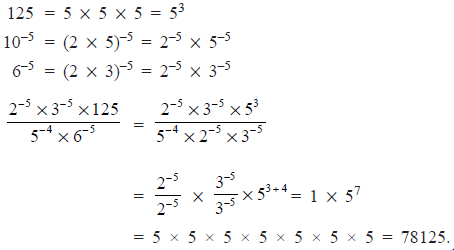Question 5: Write the following in standard form:
(i) 0.000000456 and (ii) 34500000.

Solution: We have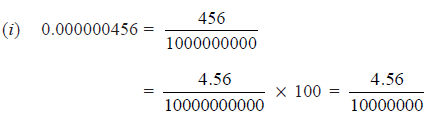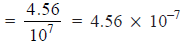Thus, 0.000000456 = 4.56 * 10–7

(ii) 34500000 = 345 * 100000
= 3.45 * 100 * 100000
= 3.45 * 102 * 105 = 3.45 * 102+5
= 3.45 * 107
Thus, 34500000 = 3.45 * 107

Question 6: Express 2.0001 * 109 in usual form.
Solution: We have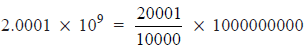= 20001 * 100000
= 2000100000
Thus, 2.0001 * 109 = 2000100000

The document Important Questions: Exponents and Powers Notes | Study Mathematics (Maths) Class 8 - Class 8 is a part of the Class 8 Course Mathematics (Maths) Class 8.
All you need of Class 8 at this link: Class 8

## Mathematics (Maths) Class 8

187 videos|323 docs|48 tests
 Use Code STAYHOME200 and get INR 200 additional OFF

## Mathematics (Maths) Class 8

187 videos|323 docs|48 tests

Track your progress, build streaks, highlight & save important lessons and more!

,

,

,

,

,

,

,

,

,

,

,

,

,

,

,

,

,

,

,

,

,

;